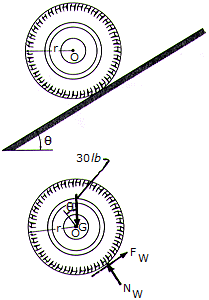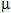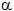# Engineering Mechanics - PKRB: Force and Animation - Discussion

### Discussion :: PKRB: Force and Animation - General Questions (Q.No.13)

13.The wheel has a weight of 30 lb, a radius of r = 0.5 ft, and a radius of gyration of kG = 0.23 ft. If the coefficient if friction between the wheel and the plane is= 0.2, determine the wheel's angular acceleration as it rolls down the incline. Set= 12°.

 [A].= 59.5 rad/s2 [B].= 8.93 rad/s2 [C].= 11.05 rad/s2 [D].= 63.3 rad/s2

Explanation:

No answer description available for this question.